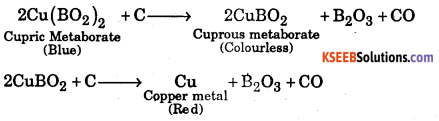# 1st PUC Chemistry Question Bank Chapter 11 The P-Block Elements

## Karnataka 1st PUC Chemistry Question Bank Chapter 11 The P-Block Elements

### 1st PUC Chemistry The P-Block Elements One Mark Questions and Answers

Question 1.
Why does carbon show maximum catenation among group 14 elements?

1. Small size of carbon atoms.
2. C-C bond energy is comparable with the bond energy between carbon and other element
3. 4 valency electrons are present in four orbitals and the tetravalency of carbon is fully saturated.

Question 2.
Name different types of charcoal.
Wood charcoal, Animal charcoal, Sugar charcoal.

Question 3.
Give one use of black diamond.
Due to their hardness, black diamonds are used in various cutting tools.

Question 4.
What type of hybridization does carbon undergo in diamond and graphite?
In diamond sp3, in graphite sp2.

Question 5.
Silicon has become a vital element in the modern electronics industry. Why?
It can function as semiconductors especially as n-type and p-type extrinsic semiconductors lubricants and insulators, Silicons are used for making water-proof papers by coating them with a thin layer of silicons.Question 6.
What is catenation?
Carbon atoms have a remarkable property of joining with one another in a large number to form a long chain and rings. This property is known as catenation or self linkage.

Question 7.
What is the technique used in preparing very pure silicon?
Zone refining.

Question 8.
Give an example for intrinsic semiconductor other than silicon.
Germanium in the pure state.

Question 9.
Who discovered fullerene 7
Richard E. Smalley, Robert F. Curl Jr. and Harold W. Kroto.

Question 10.
What kind of semiconductor is obtained when a little of phosphorus is added to silicon?
n- type semiconductor.

Question 11.
Name the oxidation states exhibited by Ge, Sn, and Pb.
+2 and +4

Question 12.
What type of semiconductor is obtained by doping germanium with indium?
p-type semiconductor

Questuon 13.
Mention the catenation capacity of Si.
16.

Question 14.
Why is boron metalloid?
Boron resembles both with metals and non-metals therefore, it is metalloid

Question 15.
Give two important ores of boron.

1. Borax, Na2B4O710H2O
2. Kemite, Na2B4O72H2O

Question 16.
Name two isotopes of boron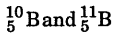are isotopes of boron.

Question 17.
Name two allotropes of boron.
Amorphous and crystalline.

Question 18.
Why does boron not form B3+ ion?
Boron does not form B3+ ion due to smallest atomic size and highest ionization energy

Question 19.
Why is crystalline boron hard solid?
It is due to strong covalent bonds.

Question 20.
Why is boron used in nuclear reactors?
Bonor can absorb neutrons.Question 21.
What is tincal ? Give its chemical formula.
Tincal (or Borax) is an ore of boron. Its formula is NaB4 Or 10H2O

Question 22.
Why does boron resemble Si?
Both have similar charge over radius ratio, i.e., similar polarizing power.

Question 23.
Why does boron form stable electron deficient compounds?
Boron has three valence electrons, it will share three electrons with other elements to form electron deficient compounds which are stable.

Question 24.
Why is boric acid (H3BO3) monobasic acid?
It accepts a pair of electrons from OH ion of H2O therefore, it is monobasic acid.

Question 25.
What type of glass is obtained when borax is added ?
Pyrex glass is a glass which is heat resistant. It can withstand high temperature.

Question 26.
What is use of diborane? Why BHs exists in form of diborane ?
BH3 exists in form of diborane (B4H6) because it is electron deficient. Diborane is used as reducing agent.

Question 27.
Complete the following
(i) B + O2
(ii) B + N2
(iii) B + Cl2
(iv) BF3 + NH2
(v) Na2B4O7+H2O →
(vi) Na2B4O7
(i) 4B + 3O2 → 2B2O3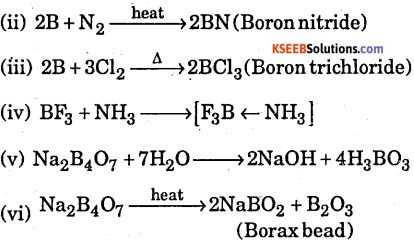Question 28.
Why does $$\mathrm{BF}_{6}^{3-}$$ not exist?
It is because ‘B’ does not have vacant d-orbitals.

Question 29.
Which type of bonds are formed by boron and why?
Boron forms covalent bonds because it cannot lose electrons or gain electron easily.

Question 30.
What is basic structural unit of orthoboric acid? Name type of bond present in them.
$$\mathrm{BO}_{3}^{3-}$$ is basic structural unit of boric acid. Covalent bonds are present.

Question 31.
Give structure of diborane.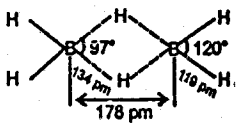Question 32.
Why do boron halides form addition compounds with ammonia and amines?
It is because they are electron deficient.

Question 33.
In which pure form does carbon exists in nature?
Fullerene

Question 34.
Give structure of CO2.
O = C = O, It has linear structure.

Question 35.
What name is given to the compounds formed by more electropositive elements with carbon?
Ionic compoundsQuestion 36.
Is carbon dioxide poisonous or not?
No, it is not poisonous.

Question 37.
What is producer gas ?
Producer gas is mixture of CO and N2 in the ratio of 2 : 1

Question 38.
Buckminster fullerene is a crystalline allotrope of which element ?
Carbon

Question 39.
What is state of hybridisation of C in $$\mathrm{co}_{3}^{3-}$$ ?
‘C’ in $$\mathrm{co}_{2}^{3-}$$ has sp2 hybridisation.

Question 40.
How does BF3 act as catalyst in industrial process?
BF3 is electron deficient, therefore acts as catalyst in industrial processes.

Question 41.
Why is BF3 weaker Lewise acid than BCl3
BF3 is weaker Lewis acid than BCl3 because of more effective bonding in case of F due to smaller size, than Cl.

Question 42.
Name the elements present in boranes.
Boranes are made up of boron and hydrogen.

Question 43.
Write the state of hybridization in BF3.
‘B’ in BF3 has sp2 hybridisation

Question 44.
Why does boric acid act as Lewis acid ?
It is because in boric acid, boron does not have its octet complete. It accepts OH- from
water in aqueous solution B(OH)3 + H2O → [B(OH)4] + H+

Question 45.
Why does boron form electron deficient compounds ?
It is because boron has three valence electrons. It shares three electrons and gets six electrons, i.e., its octet is not complete. Therefore, it forms electron deficient compounds.

Question 46.
Mention the chief reason for the anomalous behaviour of boron in group 13 of the periodic table.
It is due to small size and higher ionization energy and high charge/size ratio of boron.

Question 47.
Mention the type of hybrid orbitals of silicon in $$\mathrm{SiF}_{6}^{2-}$$ ion.
sp3d2

Question 48.
Mention the state of hybridization of B in $$\mathrm{BH}_{4}^{-}$$
sp3

Question 49.
How is that silicon atoms can have a co-ordination number more than four but carbon atoms cannot?
Silicon has vacant d-orbitals, therefore, it can have coordination number more than four but carbon cannot have because it does not have vacant d-orbitals.

Question 50.
CO2 is gas while SiO4 is solid at room temperature. State a reason for this.
CO2 exists as decrete molecules, therefore, it has weak Van der Walls’ forces for attraction whereas SiO2 is three dimensional covalent solid.Question 51.
Between AIF3 and AICl3 which one will have a higher melting point.
AIF3 is more ionic, therefore, has higher melting point.

Question 52.
Why is that aluminium metal cannot be obtained by electrolysis of an aqueous solution of a salt of aluminium?
It is because aluminium metal reacts with water at high temperature.

Question 53.
Which one of the following elements +1 oxidation state as well: Al, B, Ca, Tl, Be?
TI shows +1 oxidation acid.

Question 54.
Carbon and Silicon are mainly tetravalent but Ge, Sn and Pb show divalency. Give reason.
Ge, Sn, Pb are divalent due to inert pair effect which is not there in carbon and silicon.

Question 55.
What property of anhydrous AlCl3 makes it a very good preparative reagent in organic chemistry?
It acts as Lewis acid.

Question 56.
Although pure silicon is an insulator, then how does it behave as semiconductor on heating?
Silicon becomes semiconductor on just heating because electrons becomes free to move.

Question 57.
What is name given to elements which are neither metals nor non-metals?
Metalloids

Question 58.
What is general formula of silicones ?
R2SiO is general formula of silicones.

Question 59.
What is the oxidation state of Ni in [Ni(CO)4]?
Ni has oxidation state of zero in [Ni(CO)4]

Question 60.
What is inert pair effect ?
The pair of electrons in valence s-orbital is reluctant to take part in bond formation due to poor shielding effect of -d and f-electron in heavier elements. It is called inert pair effect due to which lower oxidation state becomes more stable than higher oxidation state in case of p-block elements.

Question 61.
How are linear silicones obtained ?
Linear silicones are obtained by hydrolysis of R2SiCl2 (chlorosilanes)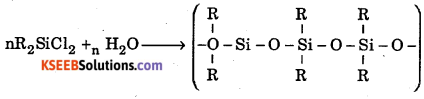Question 62.
In terms of atomic size, ionization energy and charge, explain why are ionic compounds containing B3+ not formed.
Boron has smaller atomic size, therefore, it cannot lose three electrons because its third ionization energy is very high.

Question 63.
Why does C differ from rest of elements ?
Carbon has smallest size, highest ionization energy and high electro negativity, therefore, it differs from rest of the elements.

Question 64.
What are silicates?
Silicates are minerals which consist of $$\mathrm{SiO}_{4}^{4-}$$ units arranged in different ways.

Question 65.
Write the formula of inorganic benzene.
B3N3H6Question 66.
Explain the following statement with reason.
The fullerene is considered as purest allotrope of carbon.
It is because it does not have edges, therefore, impurities cannot be absorbed on it.

Question 67.
Mention the nature of an aqueous solution of borax.
Basic in nature.

Question 68.
Boric acid is polymeric. Why?
The presence of hydrogen bonds makes it polymeric.

Question 69.
Mention the type of hybridization of boron in diborane.
Sp3

Question 70.
Which is thermodynamically most stable form of carbon ?
Graphite

Question 71.
State the trends observed in case of each of the following: Oxidation state of the elements of Group 14.
Group 14 elements show +2 and +4 oxidation states. Tendency to show +2 oxidation state increases down the group due to inert pair effect.

Question 72.
How does the boric acid polymerise ?
Through hydrogen bonding.

Question 73.
Does BH3 exists?
No.

Question 74.
How does boron interact with NaOH ?
2B + 6NaOH → 2Na3B03 +3H2

Question 75.
What is the oxidation state of C in
(a) CO
(b) HCN
(c) H2CO3
(d) CaC2.
(a) +2, (b) +2, (c) +4, (d) -1

Questuon 76.
Give two examples of electron-deficient compounds.
BF3 and B3H6.

Question 77.
Arrange the following halides of boron in the increasing order of acidic character. BF3, BCI3, BBr3, BI3.
BF3 < BCI3 < BBr3 < BI3.

Question 78.
Which form of carbon is used to decolourise sugar ?
Bone charcoal.

Question 79.
What does we get when Cone. H2SO4 is dropped on sugar ?
Sugar Charcoal.

Question 80.
What happens when a borax solution is acidified ? Write a balanced equation for the reaction.
Boric acid is formed (Na2B3O7 + 2HC1 + 5H2)O →2Nacl + 4H3BO3### 1st PUC Chemistry The P-Block Elements Two Marks Questions and Answers

Question 1.
Give the chemical reaction as an evidence for each of the following observation?

• Tin (II) is a reducing agent whereas lead (II) is not
• Gallium (I) undergoes disproportionation reaction.

• It is because Pb2+ is more stable than Pb4+ due to inert pair effect whereas Sn4+ is more stable than Sn2+. Therefore Sn2+ is good reducing agent 3Ga+ —> 2Ga + Ga3+
• It is because Ga3+ is more stable than Ga+

Question 2.
Write the chemical equation for the reactions which occur when : R2SiCl2 is subjected to hydrolysis.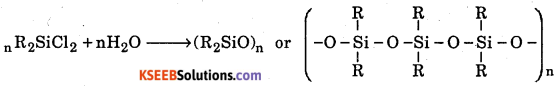Question 3.
Complete the following chemical reaction :
1. $$\mathrm{RCI}+\mathrm{Si} \frac{\text { Cupower }}{570 \mathrm{K}}$$
2RC1 + Si → R2SiCl2

Questuon 4.
Describe two similarities and two dissimilarities between B and Al.
Similarities :

1. Both have similar electronic configuration
2. Both have same number of valence electrons

Dissimilarities:

1. B is non-metal; Al is metal
2. B forms acidic oxide (B2O3) whereas Al form Al2O2 which is amphoteric

Question 5.
Draw a labelled diagram to show the dimeric structure of Al(III) chloride. State one use of anhydrous AICl3.
The structure of AICl3 is shown below.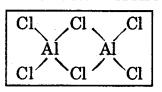It issued in preparation of organic compounds in Friedal Crafts reaction.

Question 6.
Arrange the following compounds in decreasing order of property indicated against each. Give reason for your answer : BCI3, AICl3, GaGL3, InCl3, TiCl3 (Stability of +3 oxidation state)
BCl3 > AICl3> GaCl3 > InCl3 > TiCl3 because of inert pair effect stability of +3 oxidation state decreases.

Question 7.
Discuss the diagonal relationship of Be and Al with regard to

• the action of alkali and
• the structure of the chlorides.

Be and Al resemble with each other due to same charge over radius ratio.

• Both B and A1 amphoteric oxides
• Be and A1 form covalent compounds
• BeCl2 exists as polymer whereas AICl3 exists as dimmer
• Both Be and A1 react with NaOH to form similar compounds.

Question 8.
C and Si are always tetravalent but Ge, Sn, Pb show divalency.
Ge, Sn, Pb show divalency due to inert pair effect, Pb2+ is more stable than Pb4+.

Question 9.
Which of the following is acidic and why: SiCO2, AI2O3, PbCO2, SiCO2?
SiCO2 is acidic oxide because Si is non-metal.

Question 10.
As we move down in group 13 elements increase in atomic size is comparatively very less. Explain.
It is due to poor shielding effect of d and f-electrons, as result of which effective nuclear charge increase.Question 11.
Write the chemistry involved in etching of glass.
SiO2 + 4HF → SiF4 + 2H2O
SiF4 +2HF → H2SiF6(Fluorosilicicacid)

Question 12.
Elemental silicon does not form graphite like structure as carbon does. Give reason.
It is because ‘Si’ cannot form pπ-pπ bond like carbon in graphite due to larger atomic size.

Question 13.
What are silicones ?
Silicones are rubber like polymers.

Question 14.
Give the shapes of
(a) SiF4,
(b) $$\mathrm{SiF}_{6}^{2-}$$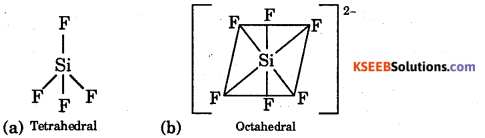Questuon 15.
Arrange the following in decreasing order of boiling point giving reason CH4, GeH4, SnH4, SiH4
SnH4 > GeH4 > SiH4 > CH4
As we go down the group from C to Sn surface area of hydrides increases therefore, Van der Waals’ force increases hence boiling point increases.

Question 16.
Explain the following: (a) CaO reacts with SiCO2 to form a slag.
(a)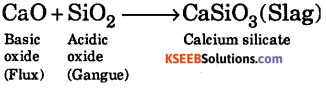### 1st PUC Chemistry The P-Block Elements Threer / Four / Five Marks Questions and Answers

Question 1.
What are allotropy ? Mention allotropy of Pb, Sn, Ge, Si.
Allotropy: The existence of same element in two or more forms in the same physical state but having different properties called allotropy. The different forms are called allotrope.
Pb : —> No allotrope exists.
Sn : —> Grey ( ) = White ( ) = ; Rhombic { Tin Cry : the sound when crystals of tin is Bent or deformed}
Ge : —> Two (Both are crystalline form.)
Si: —> Two ( Both are crystalline form.)

Question 2.
Explain the oxidation property of group 14 elements.
Group 14 elements show commonly +2 and +4 oxidation state. The carbon family also shows both positive and negative oxidation state. It shows +2 oxidation state when np2 electrons involved in the bond formation Ex-SnCl2, PbSO4. This show +4 oxidation state when ns2np2 electrons involved in the bond formation. Ex-SnCl4

Question 3.
Explain semiconductors with its types and uses.
Semiconductors are the materials their conductance is lies between those of conductors and insulators. They allow only a certain portion of the current to pass through them. Materials used for semiconductors should be extremely pure and is obtained by zone refining.

Question 4.
Explain the types of semiconductors.
1. Intrinsic Semiconductors: If Si of Ge is sufficiently heated the energy supplied breaks some of the covalent bonds and electrons become free to carry current. When the electrons are get free are released, positive holes are left behind in the lattice. In intrinsic semiconductors, the number of holes and electrons will be equal.

2. Extrinsic semiconductors: These are formed doping (addition of impurities of certain elements) to the insulators.

Question 5.
Explain the types Of Extrinsic Semiconductors
I. n-type semiconductor: Doping of (Group 14 elements) Si, Ge, with pentavalent impurities (group 15 elements) like P. As, produces excess of electron and they are called n-type semiconductors. The symbol ‘n’ indicates the flow of negative charge in them.

Question 6.
How does metallic character vary among the group 14 elements? How is it related to ionisation energy?
Carbon and silicon are non metals. Germanium is a metalloid and tin and lead are metals. Metallic character increases from carbon to lead. The change from non metallic to metallic character is due to the increase in atomic size from carbon to lead. Consequently the ionization potential decreases from carbon to lead.

Hence the electropositive character increases favouring ionic bonding especially with oxidation state +2. Whereas carbon and silicon show +4 oxidation state forming covalent bonding with electron sharing.Question 7.
Distinguish between n- type and p – type semiconductors.
n – type semiconductors are formed by doping pure Si or Ge with pentavalent elements like P, As, Sb, etc, Of the five valence electrons of the added elements, four of them form covalent bonds with Si (or Ge). The fifth electron, conducts the current.

In p – type conductor Si or Ge is doped with trivalent elements like Boron, aluminum or indium. The three valence electron of the added element form three covalent bonds with Si (or Ge) results as deficiency of one electron in the added element causes a positive hole. This type of extrinsic semiconductors are known as p – type semiconductors ( p – stands for positive hole).

Question 8.
How do you account for the tetravalency of carbon even though there are only 2 unpaired electrons ?
Electronic configurations of carbon atom in the ground state is $$1 \mathrm{s}^{2} 2 \mathrm{s}^{2} 2 \mathrm{P}_{2}^{1} 2 \mathrm{P}_{2}^{1} 2 \mathrm{P}_{2}^{0}$$ During the formation of a compound the activation energy of the reactants excites the electrons to give the configuration as $$1 \mathrm{s}^{2} 2 \mathrm{s}^{1} 2 \mathrm{p}_{\mathrm{x}}^{1} 2 \mathrm{p}_{\mathrm{y}}^{1} 2 \mathrm{p}_{\mathrm{z}}^{1}$$ Then four orbitals each containing an impaired electron, mix together and form four hybridized sp3 orbitals directed from the carbon atom tetrahedrally. Hence carbon exhibits tetravalency.

Question 9.
How do the conductivity or semic conduction can be increased?
As the temperature increases, the number of covalent bonds ruptured in an intrinsic semiconductor increases. This results in the increase of the electron hole pairs. As a result, the conductivity of the intrinsic semiconductor increases with rise in temperature. When electron field is applied across these materials electron migrate in one direction and the positive holes migrate is the opposite direction and thus the material conducts. At a given temperature the conductivity of an intrinsic semiconductor is constant.

Conductivity of a semiconductor can be increased by adding either pentavalent or trivalent elements. The elements added to intrinsic semiconductors to increase conductivity are called dopants, the process of addition of dopants (impurities) is called doping. The dopped semiconductors are called extrensic Semiconductors.

Question 10.
Write a note on structure of Fullerene. Mention any two of its application.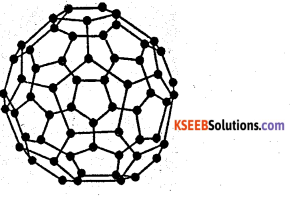Richard E. Smalley; Robert F. Curl Jr and Sir Harold W. Kroto the discoverers of this new form of pure carbon laked fullerene, they shared the 1996 Nobel Prize in chemistry for their discovery.

This third crystalline form of carbon discovered was named as buckminster fullerene which is commonly called fullerenes. They were named so because their structure resembles geodestic domes designed by the American scientist, engineer and philosopher R. Buckminster Fuller, They are also philosopher R. Buckminster Fuller. They are also nicknamed “Bucky ball” because their shape resembles a soccer ball.

Fullerenes are large spheroidal Cn molecules with n = 60 or more. When an electric arc is struck between graphite electrodes in an inert atmosphere a large quantity of soot is formed together with a significant quantity of C60 and much smaller quantities of inter related fullerenes such as C70 ; C76 ; C78.

When graphite is vapourised using a high power laser, fullerene C60 is obtained. The fullerene C60 bucky ball, has the same form as in soccer ball. In this each carbon atom is sp2 hybridised. Each carbon atom has three neighbouring carbon atoms and forms two single and a double bond. C60 has 32 faces. 20 of which are hexagons and 12 of which are pentagons. Each pentagon is surrounded by five hexagons and each hexagon is linked to three pentagons and each three hexagons. It has 60 vertices with a carbon atom at each vertex.

Question 11.
Write the characteristic uses of fullerene.
C60 dissolves in hydrocarbon solvents such as hexane, benzene, toluene etc giving magenta solutions. Fullerenes are the least stable of the carbon allotropes and graphite is the most stable. Fullerenes react with alkali metals to produce solids having composition such as K3 C60. This compound acts as a superconductor below 18K. Fullerene C114 has more surface area and can withstand a very high temperature. Hence it can be used as a lubricant in satellites. Fullerenes are used as industrial catalyst and as lubricant in the treatment of cancer.Question 12.
Explain the structure of graphite giving the reason for its softness as well as its electrical conductivity.
Good conductor of electricity : In. graphite carbon atom is sp2 hybridised. Out of four valiancy electrons in each carbon atom only three electrons, one from 2s and two from 2p are involved in hybridisation. One spare electron in its 2pz orbital is left free. These 2pz orbital overlap to form delocalised π system which extends above and below each layer. In this delocalised π electrons are free to move within the layer and hence graphite is a good conductor of electricity.

The conductivity of graphite perpendicular to the plane of the layers of hexagons is low and increases with increase in temperature, signifying that graphite is a semiconductor in that direction. The electrical conductivity is much higher parallel to the plane but decreases as the temperature is raised.

Soft, flaky and slippery substance : In graphite each layers of hexagon are held together by a weak Vander Waal’s force of attraction at a large distance of 3.40 Å. Even the slight pressure causes the layers to slide over one another. Hence graphite is soft, flaky and slippery. Graphite is used as a lubricant.

Question 13.
Explain the structure of diamond giving the reason for its hardness as well as non – conductivity of electricity.
Non-conductor of electricity: All the four electrons present in the valence shell of carbon atoms are used up in sp3 hybridisation and form sigma bonds. No mobile electrons are left in the diamond crystals to allow the conduction of electricity. Hence diamond is a non-conductor of electricity.

Extremely hard: Due to high strength of covalent bonds holding all the carbon atoms in diamond together, diamond is very hard. Infact, diamond is the hardest substance known.

Question 14.
Give the difference in structure of the following pair of compounds : CO2 and SiO2.
CO2 linear molecule and exists as monomer. It is gas while SiO2 is solid at room temperature due to three dimensional network in which each Si atom is covalently bonded to four oxygen atoms tetrahedrally. In CO2, ‘C’ is sp-hybridised while in SiO2, ‘Si’ is sp3 hybridised. CO2 is discrete molecule where as SiO2 is 3-dimensional solid.

Question 15.
Explain the formation of

(i) water gas
(ii) producer gas. Give their uses.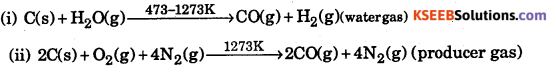Water gas and producer gas are used as fuel

Question 16.
What happens when CO2 is passed through lime water
(i) for short duration
(ii) for a long duration ?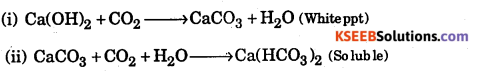Question 17.
The first element in every group of representative elements shows properties different from the characteristic properties of the group. Name three such elements and mention two abnormal properties of each one of them.
Boron carbon Nitrogen
Boron

• W, forms acuhc. oxides -whereas owners item. am-phofork and.bask, oxide.
• It cannot form [BFe]3′ whereas others can form such type of complexes.

Carbon:

• It shows property of catenation to maximum extent.

Nitrogen

• N2 is gas others are solid
• NH2 is liquid, other hydrides are gases.

Question 18.
Give equations for the following:

• Preparation of CO2,
• Basic properties of Na2CO3,
• Formation of acetylene.

• CaCO3 + 2HC1 —> CaCl2 + CO2 + H2O
• Na2CO3 + 2H20 —> 2Na0H + H2CO3
• CaC2(calcium carbide) + 2H20 —>Ca(OH)2 + C2H2(Acetylene)

Question 19.
What is dry ice ? Why is it called so? How will you prepare pure sample of CO (Carbon monoxide)?
Solid CO2 is called dry ice. It is called dry ice because it directly changes into gaseous state without becoming liquid.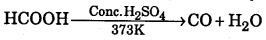Question 20.
What happens when ?

• Quick lime is heated with coke ?
• Carbon monoxide reacts with Cl2?
• Plants absorb CO2?

• CaO + 3C —> CaC2(CalciumCarbide) + CO,
• CO + Cl2 —> COCl2 (Phosgene)
• 6CO2 + 6H2O —> C6H12O6(glucose) + 6O2Question 21.
Write balanced equations for the following reactions and name the main product formed in each case,

• NaBH4 +I2 —>
• B2h6 +NaH —>
• $$\mathrm{BF}_{3}+\mathrm{LiH} \stackrel{450 \mathrm{K}}{\longrightarrow}$$
• SiCl4 + H2O —>

• 2NaBH4 +I2 —> 2Nal + +H2 + B2H6 (Diborane)
• B2H6+2NaH —> 2NaBH4 (sodium borohydride)
• 2BF3 + 6LiH —> 6LiF + B2H6 (Diborane)
• SiCl4 + 2H2O —> 4HCl + Si(OH)4 (Silicicacid)

Question 22.
Give a comparative account of the chemistry of carbon and silicon with regard to their

• property of catenation and
• stability of hybrids and oxides.

• Carbon shows property of catenation to more extent than silicon due to small size and tendency to form pπ-pπ multiple bonds with itself
• Stability of hybrids : CH4 is more stable than SiH4 due to small size of ‘C’.
• CO4 is gas whereas SiO2 is three dimensional covalent solid, therefore highly stable as compared to CO2.

Question 23.
A certain salt X, gives the following results.
(i) Its aqueous solution shows alkaline to litmus paper
(ii) It swells up to a glassy material Y on strong heating
(iii) When cone H2SO4 is added to a hot solution of X, white crystal of an acid Z separates out-Write equations for all the above reactions and identify X, Y and Z.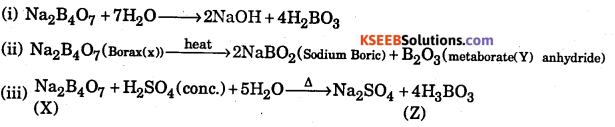Question 24.
Write balanced equations for:

1. BF3 + LiH →
2. B2H6 + H2O →
3. NaH + B2H6
4. $$\mathrm{H}_{3} \mathrm{BO}_{3} \stackrel{\Delta}{\longrightarrow}$$
5. A1 + NaOH →
6. B2H6 + NH3

1. 2BF3 +6LiH →B2H6 +6LiF

2. B2H6 + 6H2O → 2B(OH)3 + 6H2

3. 2NaH + B2H6 → 2NaBH4

4. $$\begin{array}{l}{\mathrm{H}_{3} \mathrm{BO}_{3} \stackrel{\Delta}{\longrightarrow} \mathrm{HBO}_{2}+\mathrm{H}_{2} \mathrm{O}} \\ {4 \mathrm{HBO}_{4} \stackrel{\Delta}{\longrightarrow} 2 \mathrm{B}_{2} \mathrm{O}_{3}+2 \mathrm{H}_{2} \mathrm{O}}\end{array}$$

5. Al + 3NaOH → A1(0H)3 +3Na

6. $$3 \mathrm{B}_{2} \mathrm{H}_{6}+6 \mathrm{NH}_{3} \stackrel{\text { heat }}{\longrightarrow} 2 \mathrm{B}_{3} \mathrm{N}_{3} \mathrm{H}_{6}+12 \mathrm{H}_{2}$$

Question 25.
Give one method for industrial preparation and one for laboratory preparation of CO and CO2 each.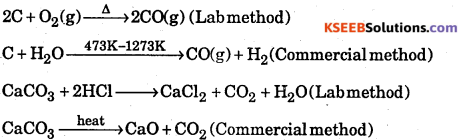Question 26.
(a) Carbon dioxide is non-polar while water is polar. What conclusion do you draw about their structures from these.
(b) Classify the following compounds into acidic, basic and amphoteric oxides. Al2O3, Cl2O7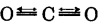is linear, bond moments are equal and opposite, net dipole moment is zero. Water is bent molecule, it has net dipole moment .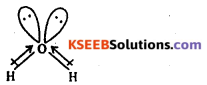Al2O3 is amphoteric where Cl2O7 is acidic oxide.

Question 27.
A white crystalline solid ‘A’ dissolves in water to give an alkaline solution. On heating ‘A’ first loses water molecules and swells up. On further heating it turns into a transparent liquid which solidifies into a glassy bead.
‘A’ is Borax i.e. Na2[B4O5(OH)4].8H2O which is also written as.
The reactions are: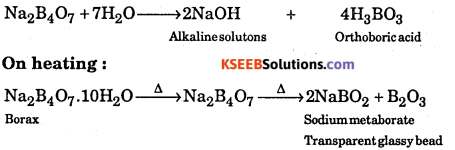Question 28.
Boric acid is a weak acid. It acts as an acid only on first receiving OH- from the medium and then releasing H+ ions. From the following organic compounds; select the one in presence of which it acts as a strong acid. Glycerol, Ethylene, Ethylalcohol, Acetic Acid.
It acts as a strong acid in presence of glyceol.
Reason: Acetic acid itself is an acid and cannot give OH ion, ethyl alcohol being a primary alcohol is alos acidic. Ethylene also can’t furnish OH ion. But glycerol that has 3-OH groups. One of which is a secondary -OH group can assist boric acid to release H+ ions.Question 29.
A compound ‘X’ or reduction with LiAH4 gives a hydride ‘Y that contains 21.72% hydrogen along with other products. The compound ‘Y’ reacts with air explosively resulting in boron trioxide. Identify ‘X’ and ‘Y’. Give balanced reactions involved in the formation of ‘Y’ and its reactions with air. Draw the structure of Y.
“X’ is BCl3 Boron Trichloride Y is B2H6 as percentage of hydrogen given is 21.72%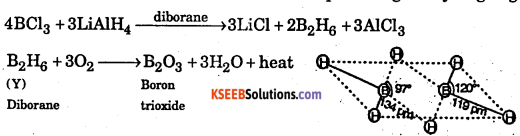Question 30.
Mention three important uses of borax.

• as a flux soldering and welding industry
• in the manufacture of borosilicate glass (or pyrex glass)
• in making enamels and glazes
• in stiffening of candle wicks
• in softening of water
• in qualitative analysis for borax bead test in laboratory.

Question 31.
Mention some important properties of carbon monoxide.
1. It is colourless, odourless gas, slightly soluble in water.

2. It is highly poisonous. It combines with haemoglobin in the red blood cells to form carboxy-haemoglobin which cannot absorb oxygen and thus supply of oxygen to the body is reduced.

3. It burns with a pale blue flame forming CO2; i.e., 2CO + O2 → 2CO2

4. It is a reducing-agent. It reduced some metal oxides into metal,
Fe2O3 + 3CO → 2Fe + 3CO2

5. It combines with transition metals like iron, cobalt, nickel to form their carbonyl compounds;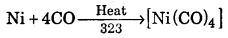Question 32.
What are halides of carbon ? Give few examples.
Carbon combines with halogens to form both simple and mixed tetrahalides. In case of simple halides all the four expected tetrahalides (e.g. CF4, Cl4, CBr4 and Cl4) are known to exist. The stability of the simple tetrahalides (CF4 > CCl4 > CBr4 > Cl4)
Amongst the mixed halides the better known compounds are
(CFCl3,CF2Cl2 and CCl3Br)

Question 33.
What is allotropy ? Give examples of allotropes.
Two or more forms of the same elements in same physical state which differ in their physical properties but have same chemical properties are called allotropic forms or (allotropes) and the phenomenon is called allotropy.
Carbon, phosphorus and sulphur are some elements which exhibit allotropy.

• Diamond and graphite are allotropic forms of carbon
• Red phosphorus and white phosphorus are allotropes of phosphorus.
• Rhombic sulphur, monoclinic sulphur and plastic sulphur are allotropic forms of sulphur.

Question 34.
Draw the structure of
(a) anion cyclic silicates
(b) anion sheet silicates.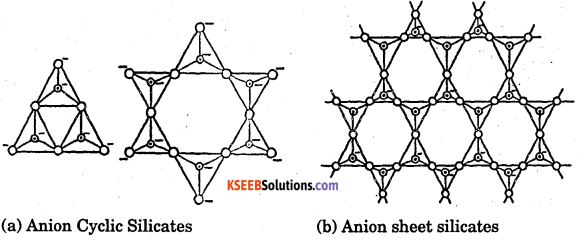Question 35.
Give three uses of different allotropic forms of carbon.

 Forms of carbon Uses Diamond Gemstone, cutting, drilling, grinding, polishing, industry. Graphite Reducing agent, refractories, pencils, high temperature, crucibles, electrode making, moderator in nuclear reactors, high strength composite materials. Activated carbon Rubber industry, pigments in ink, paints and plastics Coke Fuel, strut manufacture Charcoal Fuel, reducing agent, Adsorption.

Question 36.
Write balanced equation for the following:
(i) BF3 + LiH →
(ii) B2H6+H2O →
(iii) NaH + B2H6
(iv) H3BO3
(v) A1 + NaOH + H2O →
(vi) B2H6 + NH3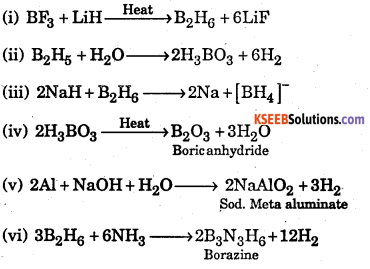Question 37.
Draw the structure of fullerene C60Question 38.
Draw the structure of graphite.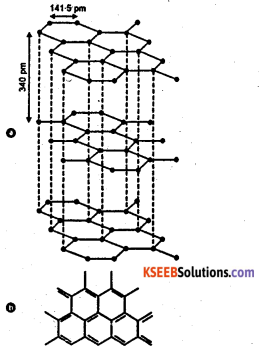Question 39.
How does electron deficient compound BF3 achieve electronic saturation i.e., the fully occupied outer electron shells ?
1. By multiple bonding, e.g., BF3 in which a lone pair of electrons of each fluorine atom may be used in a B → F π-bond (back bonding) involving the vacant orbital on the boron atom.

2. By formation of complexes in which electrons are received from a donor molecule, e.g., F3B → NH3. Boron compounds, thus, behave as Lewis acids.

Question 40.
Compound X on reduction with LiAH4 gives a hydride Y containing 21.72% hydrogen along with other products. The compound Y reacts with air explosively resulting in boron trioxide. Identify X and Y. Give balanced equations involved in the formation of Y and its reaction with air. Draw the structure of Y.
Step 1: To determine the molecular formula and structure of compound Y.
1. Since the hydride Y reacts with air forming boron trioxide, therefore, Y must be an hydride of boron.
2. %H = 21.72% (Given)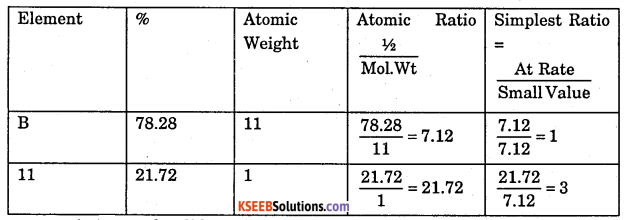∴ Emperical formula of Y = BH3
Since boron forms two types of hydrides, i.e., BnHn+4 (nidoboranes) and BnHn+6 (arachnoboranes), therefore, Y must be a nidoborane with n = 2. Thus, M.F of Y = B2H6 . If Y is B2H6 (diborane), then its structure must be as follows :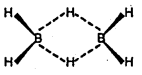Bridges B…H = 134 pm Terminal B – H = 119 pm

Step 2: To determine the structure of the compound X.
Since compound Y i.e., B2H6 is formed by reduction of compound X with LiAH4 ,
therefore, X must either BCl3 or BF3.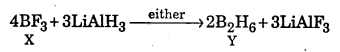The equation representing the reaction of Y with O2 may be written as follows :
B2H6 + 3O2 → 2B2O3 + 3H2O
Diborane,Y
Thus X = BF3 and Y = B2H6

Question 41.
How is boron obtained from boran? Give chemical equations with reaction conditions and its reaction with HCl1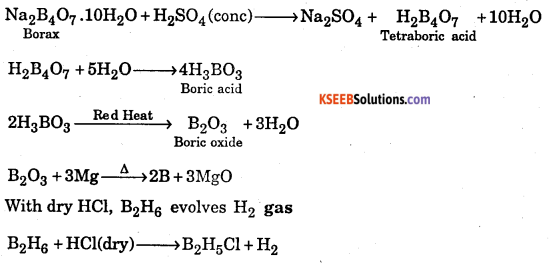Question 42.
Which one is more soluble in diethyl ether, anhydrous AlCl3 or hydrous AICl3 ? Explain in terms of bonding ?
Answer: An hydrous AICI3 is an electron-deficient compound while hydrated AICI3 is not. Therefore, anhyd. AICI3 is more soluble in diethyl ether because the oxygen atom of either donates a pair or electrons to the vacant p-orbital on the A1 atom in AICI3 forming a coordinate bond.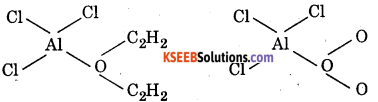In case of hydrated AlCl3. Al is not electron deficient since H2O has already donated a pair of electrons to it.

Question 43.
Mention the structures of (CH3)3N and (Me3Si)3 N. Are they isostructural? Justify your answer.
Answer: (CH3)3 N is pyramidal involving sp3. hybridisation of N atom. Nitrogen in (Me3Si)3 is however, sp2 -hybridized. The reason being that the p-orbit of N containing the lone pair of electrons overlaps with a vacant d-orbital of silicon. As a result of this pπ – dπ back bonding, (Me3Si)3 N is planar.Question 44.
1. Describe with equation what happens when carbon is heated with cone. H2SO4 ?
2. State how will you separate C02 and S02 from the mixture?
1. H2S04 oxidies C to CO2 and itself is reduced to SO2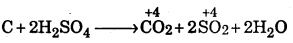2. Since the O.N. of C is CO2 is +4 which is the maximum for C, therefore, it cannot act as a reducing agent. In contrast the O.N. of S is SO2 is also +4 but its maximum. O.N. +6, therefore, it can act as reducing agent. This reducing gas can be removed by passing the mixture of these gases through acidified K2Cr2O7 solution when orange solution turns green due to reduction of $$\mathrm{Cr}_{2} \mathrm{O}_{7}^{2-}$$ ion to Cr3+ ions while CO2 passes out unreacted.

Question 45.
What is inorganic benzene ? Why is it so called ? How will you get it from diborane?
Borazine or borazole (B3N3H6) is known as inorganic benzene. It is also called Because the structure of borazine is similar to that of benzene.
It is also isoelectronic and isosteric with benzene.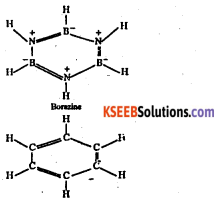Like carbon is benzene, both N and B in borazine are sp2 hybridized. Each N has a p-orbtal which is perpendicular to the a-bonding orbitals and contains a lone pair of electrons. In contrast, each B has an empty p-orbitals which is also perpendicular to the plane of the ring. Thus, the x-bonding in borazine is dative and it arises from the sideways overlap of fully filled orbitals of N and empty p-orbitals of B.

Preparation of borazine : At low temperature, diborane combines with ammonia to form an addition compound.
$$\mathrm{B}_{2} \mathrm{H}_{6}+2 \mathrm{NH}_{3} \stackrel{\text { Low Temp. }}{\longrightarrow} \mathrm{B}_{2} \mathrm{H}_{6} \cdot 2 \mathrm{NH}_{3}$$
When this addition compound is heated to 473 K, it decomposes to form a volatile compound called borazine.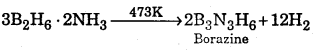Question 46.
State with equations what happens when borax is heated on a platinum wire loop and to the resulting transparent mass a minute amount of CuO is added and the mixture is again heated first in the oxidizing flame and then in the reducing flame of a Bunsen Burner ?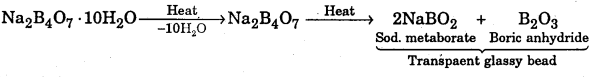$$\mathrm{CuO}+\mathrm{B}_{2} \mathrm{O}_{3} \frac{\text { oxidising }}{\text { flame }} \mathrm{Cu}\left(\mathrm{BO}_{2}\right)_{2}$$(Cupric metaborate (blue)